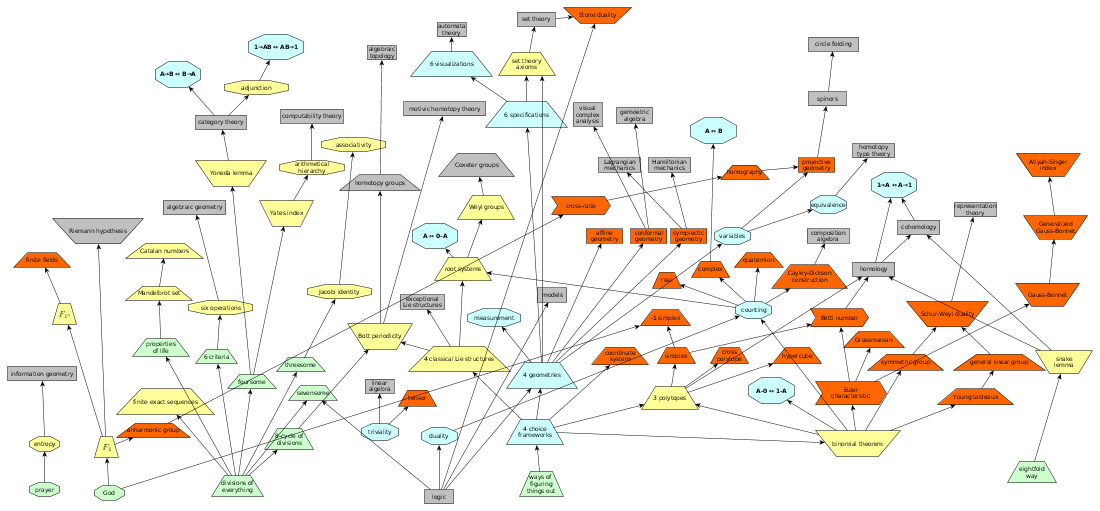# Book: MathNotebook

I'm starting to write up the results that I'm getting as I investigate the root of mathematics.Current research program

• Relate the four classical Lie families to the four levels (metalogics, geometries) in the ways of figuring things out.
• Understand the four classical Lie families (the choice frameworks, root systems, geometries).
• Understand the most fundamental Lie groups {$A_2$}, then {$A_n$}, and then the other classical families.
• Understand the four levels (metalogics, geometries) in the ways of figuring things out.
• Understand the ways of figuring things out in physics.
• Understand the role of measurement in physics, both relative and absolute.
• Understand tensor products.
• Understand the significance of the foursome in mathematics and how it relates to the four levels.
• Relate the foursome and the Yoneda lemma.
• Understand Yates index theorem as a statement about the foursome, and relate it to the Yoneda lemma.
• Consider whether and how {$F_1$} models four stages of God's going beyond himself.
• Understand groups as analogues of Hopf algebras.
• Understand finite fields and what {$F_{1^n}$} could mean.
• Consider these four stages as four interpretations of the variable q in Gaussian binomial coefficients. Relate that to my theory of variables.
• Relate Bott periodicity and the eight-cycle of divisions of everything.
• Understand the Snake lemma as the eightfold way.
• Solved: Defining geometry
• How is one dimension embedded in other dimensions?
• What is a line segment? What makes it "straight"?
• What is a circle?
• What does it mean for figures to intersect?
• Can a line intersect with itself?
• Investigation: Map out the main ideas of geometry.
• Challenge: Relate the definition of geometry as "the regularity of choice" with Grothendieck's machinery.
• Investigation: What are the four geometries?
• Study: What is projective geometry all about?
• What does projective geometry say about the existence of infinity?
• Do all lines (through plane) meet at infinity at a common point? Or at a circle? Or do the ends of the lines not meet? Or do they go to a circle of infinite length?
• Study: Understand the basics of symplectic geometry.
• Compare the four geometries.
• Express the four geometries in terms of symmetric functions.
• Consider how infinity, zero and one are defined in the various geometries. How do these concepts fit together?
• How do they involve the viewer and their perspective? How might that relate to Christopher Alexander's principles of life and the plane of the viewer?
• How are they related to the twelve topologies?
• Investigate orientation. What is the notion of orientation for points, lines, etc? grounding different geometries? considering building spaces bottom up (adding lines) and removing spaces top down (removing hyperplanes)?
• Compare building spaces bottom up or by deletion top down with choices left or right, etc.
• Study Norman Wildberger's book and videos as an expression of geometry and try to express it all systematically, for example, using symmetric functions.
• List out the results of universal hyperbolic geometry and state them in terms of symmetric functions.
• Understand algebraic geometry (sheaves, etc.) by analyzing its theorems.
• Understand Bott periodicity.
• Relate Bott periodicity to the eight divisions of everything and to the three operations.
• Using complex numbers, interpret {$d/dz \: e^z$}
• Study the Catalan numbers and the Mandelbrot set
• Check what happens if I plug in different values into the Catalan power series.
• What is a combinatorial interpretation of P - P(n), the generators of the Mandelbrot set, in terms of the Catalan numbers? Get help to generate the difference. What is the best software for that?
Parsiųstas iš http://www.ms.lt/sodas/Book/MathNotebook
Puslapis paskutinį kartą pakeistas 2019 spalio 09 d., 21:35